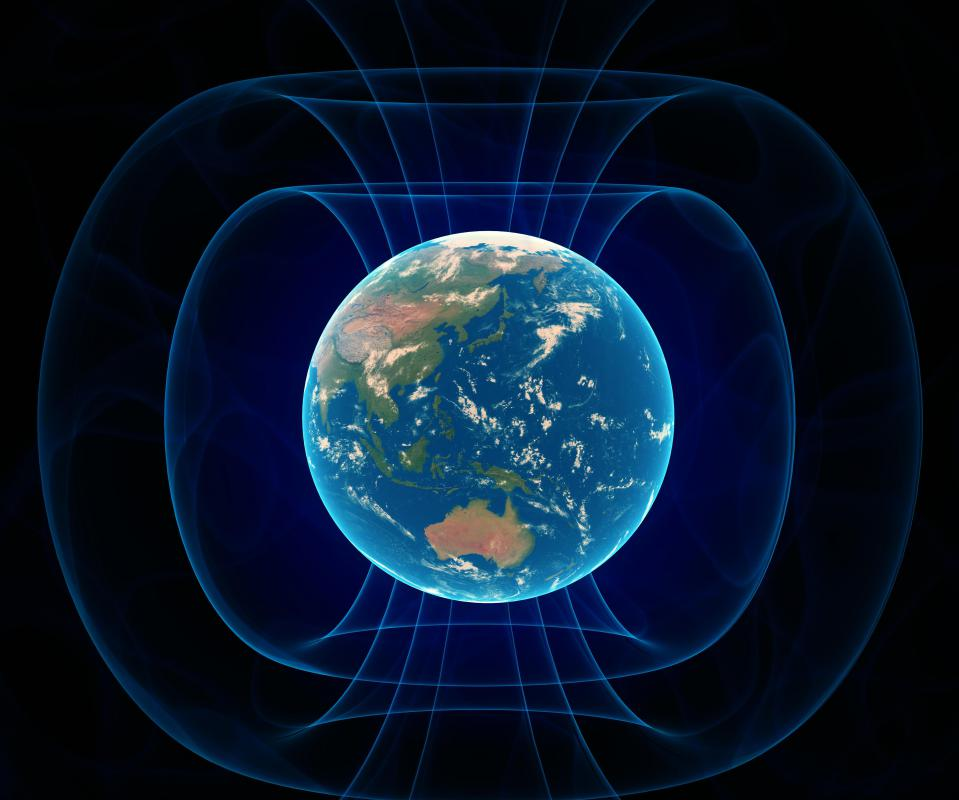# What Is a Tangent Galvanometer?

Andrew Kirmayer

Electric current is often measured using an instrument called a tangent galvanometer. Able to measure the presence as well as the direction and power of currents, the instrument was first used in the early 1800s. It typically has a vertical copper wire coil, wrapped around a circular frame, and a compass in the middle. The compass needle generally responds to the magnetic field of the electrical current, which is compared to the Earth’s magnetic field in the experiment. This scientific instrument has been built in many forms and more modern ones often use beams of light to determine measurements, while some versions are used to measure the magnetic field of the EarthSome tangent galvanometers are used to measure the Earth's magnetic field.

The instrument works based on the tangent law of magnetism. This principle defines the tangent of the angle, traveled through by the compass needle, as being proportionate to a ratio of how strong two magnetic fields are. These fields are usually perpendicular to one another. Currents measured are typically proportional to the tangent of the same angle the needle goes through.

A tangent galvanometer is usually placed on a flat surface and the compass is used to align the coil north to south. The needle of the compass is then typically lined up with the direction of the coil so, when current is applied, the angle the needle turns in can be measured. On a tangent galvanometer, the compass needle can rotate in any horizontal direction. A scale on the device has four quadrants, each equaling a quarter of a circle, while a pointer attached to the needle at a right angle can provide a means to read the scale and take measurements.

Most tangent galvanometers have a single ring, but some kinds have two rings placed side by side. The distance between the rings can vary depending on the instrument. For an optimal magnetic field, however, the distance between them should be the same as the coil radius.

One design problem of the tangent galvanometer has often been the need to have a short needle that does not dampen the Earth’s magnetic field. Small current changes are often hard to read if the needle is too short, so some modern types of tangent galvanometer can use a hollow one attached to a mirror. When light is aimed towards the mirror, it can pass through the needle and shine on the scale to make small changes in position evident during a tangent galvanometer experiment.

## You might also Like